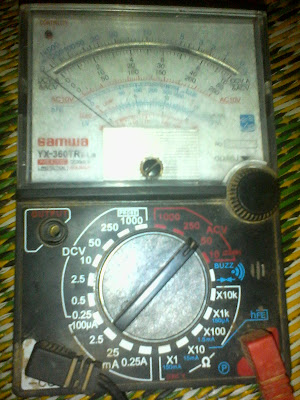# What is a Multimeter? and It's Uses

This post is about " what is a multimeter or the function of multi meter, in this post we discus working of multi meter shortly and after this post we will complete write about the multimeter uses.

## What is a Multimeter and it's Function

Multimeter is a electronic measurement device which we use for testing Ohm or Resistance (Ω) , AC voltage, DC voltage, Diode testing or buzz extra extra.
There are two types of mulitimeter which are Analog and Digital. A analog multimeter have moving pointer which move over a scale. A Digital multimeter display the measurement value in numbers. The advantage of a multi meter is that we can use for different measurements.
E.g We can use it for DC voltage testing, we can use for Ohm (Ω) or Resistance testing, We can check the AC voltage.

Here is a look of Digital and Analog Multimeter.Analog MultimeterDigital Multimeter

This post is a short note about multi meter and know you understood that what is a multimeter and it's function. How ever i will soon write about uses of multimeter with complete guide.